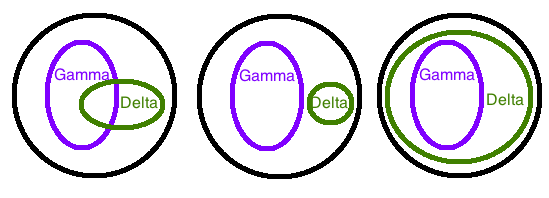# Representation - propositional logic

We begin our course by studying propositional logic. After we introduce the definitions, we will discuss satisfiability in propositional logic and then move on to state of the art techniques to solve systems specified with propositional logic.

# Definitions

Propositional logic is a formal language used to specify knowledge in a mathematically rigorous way. We first define the syntax (grammar) and then the semantics (meaning) of sentences in propositional logic.

## Syntax

We denote our propositional variables by $p_{1},...,p_{n}$ where each $p_{i}$ is a binary variable that can be true or false.

Sentences are defined recursively as follows:

• A propositional variable is a sentence (this is known as an atomic sentence)
• If $\alpha$ and $\beta$ are sentences, then $\neg\alpha$, ${\left(\alpha\lor\beta\right)}$, and ${\left(\alpha\land\beta\right)}$ are valid sentences

For example, ${\left({\left({\alpha}\lor\beta\right)}\land{\alpha}\right)}$ is a valid sentence while ${\left({\alpha}\lor\beta\land{\alpha}\right)}$ isn’t a valid sentence.

## Semantics

We have to define the notion of a world. A world ${\omega}$ is an assignment of a truth value to each propositional variable. Note that there are $2^{n}$ possible worlds if we have $n$ propositional variables.

We determine if a sentence ${\alpha}$ is true in a world ${\omega}$ using the following recursive rules:

• ${\omega}\models p_{i}$ if and only if ${\omega}(p_{i})$ is true
• ${\omega}\models\neg{\alpha}$ if and only if ${\omega}\not\models{\alpha}$
• ${\omega}\models{\left({\alpha}\lor\beta\right)}$ if and only if (${\omega}\models{\alpha}$) OR (${\omega}\models\beta$)
• ${\omega}\models{\left({\alpha}\land\beta\right)}$ if and only if (${\omega}\models{\alpha}$) AND (${\omega}\models\beta$)
• (Note that ${\alpha}\Rightarrow\beta$ is syntactic sugar for $\neg\alpha\lor\beta$, and we can use the rules above)

For example, ${\left({\alpha}\lor\neg{\alpha}\right)}$ is true in any world while ${\left({\alpha}\land\neg{\alpha}\right)}$ is never true in any world.

## Knowledge Base

A knowledge base is a collection of sentences ${\alpha}_{1},{\alpha}_{2},...,{\alpha}_{k}$ that we know are true, i.e. ${\alpha}_{1}\land{\alpha}_{2}\land...\land{\alpha}_{k}$. The sentences in the knowledge base allows us to compactly specify the allowable states of the world. By adding new sentences, the number of possible worlds consistent with our knowledge base could decrease (if we gain new information), go to zero (if the new sentence is inconsistent with the existing knowledge base), or remain the same (if the new sentence is entailed by existing sentences).Figure: By adding $\Delta$ to our KB $\Gamma$, the number of possible worlds can (i) decrease, (ii) go to zero, or (iii) remain the same.

We will make use of the following definitions:

• A sentence is satisfiable (consistent) if there exists at least one world that makes the sentence true, i.e. $Models({\alpha})\ne\phi$.
• A sentence is valid if the sentence is true in every possible world, i.e. $Models({\alpha})={\Omega}=\{0,1\}^{n}$.
• Two sentences are equivalent if they are true in the same models, e.g. ${\alpha}\lor\beta$ and $\beta\lor\alpha$.

# Satisfiability

## Inference reduces to satisfiability

The basic inference problem in the propositional logic system is the following: given the knowledge that we have about the world, is it the case that some property must always hold? In other words, what conclusion can we reach about some other sentence? The three possible cases that could result are always true, depends, and always false.

Inference problem: Does ${\alpha}\Rightarrow\beta$? We can solve this by checking if ${\alpha}\land\neg\beta$ is satisfiable.

1. If not satisfiable, then we know that ${\alpha}\Rightarrow\beta$. This is similar to the idea behind proof by contradiction.
2. If satisfiable, then there are two possible cases. Check if ${\alpha}\land\beta$ is satisfiable. If ${\alpha}\land\beta$ is satisfiable, then there is no definitive answer. If ${\alpha}\land\beta$ is not satisfiable, then we know that ${\alpha}\Rightarrow\neg\beta$.

Many problems across different domains can be encoded using propositional logic. Inference in this space basically boils down to checking satisfiability. For examples, problems such as solving Sudoku puzzles, hardware verification, and planning can be encoded as satisfiability problems and then solved efficiently using state of the art satisfiability solvers. Since a lot of research has gone into improving general SAT solvers, if some domain-specific problem has an efficient transformation into an instance of satisfiability checking, then SAT solvers can often solve these domain-specific problems efficiently.

## Conjunctive normal form

To check the satisfiability of a sentence, the first step is to convert sentences into normal form which is easier for satisfiability solvers to handle. A sentence is in conjunctive normal form (also known as clausal norm form or CNF) if a sentence is a conjunction of disjunctions.

Given any formula, we can always convert it into CNF using the following procedure:

1. The first step is to remove syntactic sugar, e.g. change ${\alpha}\Rightarrow\beta$ into $\neg{\alpha}\lor\beta$. After this step, we should only have negations, conjunctions, and disjunctions.
2. Push negations in. Negations should only appear in front of propositional variables. For example, change $\neg\neg{\alpha}$ into ${\alpha}$ and $\neg({\alpha}\lor\beta)$ into ${\left(\neg{\alpha}\land\neg\beta\right)}$.
3. Distribute disjunctions over conjunctions, e.g. change ${\alpha}\lor(\beta\land\gamma)$ into $({\alpha}\lor\beta)\land({\alpha}\lor\gamma)$. (Note that converting a sentence which is composed of many disjunctions of conjunctions into CNF using this procedure can create an exponential number of clauses.)

Checking if an assignment satisfies a CNF formula is easy. A clause is satisfiable if and only if at least one of the literals (a variable or the negation of a variable) is set to true. A sentence is satisfiable if and only if each clause is satisfiable.

Suppose we want to test the satisfiability of a formula $\Delta$ in CNF. Consider what happens when we set a propositional variable $P$ to true. If P does not belong to a given clause, then that clause is unaffected. If P belongs to a given clause, then that clause becomes satisfied and we can remove that clause from the formula. The resulting formula can be expressed as $\Delta\vert P=\{\alpha\backslash\{\neg P\}\mid\alpha\in\Delta,P\notin\alpha\}$.

Representation - propositional logic - Volodymyr Kuleshov